# Search by Topic

Filter by: Content type:
Age range:
Challenge level:

### There are 63 results

Broad Topics > Numbers and the Number System > Patterned numbers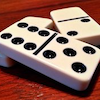### Domino Sets

##### Age 7 to 11 Challenge Level:

How do you know if your set of dominoes is complete?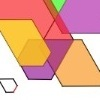### The Numbers Give the Design

##### Age 7 to 11 Challenge Level:

Make new patterns from simple turning instructions. You can have a go using pencil and paper or with a floor robot.### Tower of Hanoi

##### Age 11 to 14 Challenge Level:

The Tower of Hanoi is an ancient mathematical challenge. Working on the building blocks may help you to explain the patterns you notice.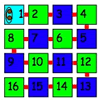### Caterpillars

##### Age 5 to 7 Challenge Level:

These caterpillars have 16 parts. What different shapes do they make if each part lies in the small squares of a 4 by 4 square?### Harmonic Triangle

##### Age 14 to 16 Challenge Level:

Can you see how to build a harmonic triangle? Can you work out the next two rows?### Hundred Square

##### Age 5 to 7 Challenge Level:

A hundred square has been printed on both sides of a piece of paper. What is on the back of 100? 58? 23? 19?### How Many Miles to Go?

##### Age 11 to 14 Challenge Level:

How many more miles must the car travel before the numbers on the milometer and the trip meter contain the same digits in the same order?### Sixty-seven Squared

##### Age 16 to 18 Challenge Level:

Evaluate these powers of 67. What do you notice? Can you convince someone what the answer would be to (a million sixes followed by a 7) squared?### Sept 03

##### Age 11 to 14 Challenge Level:

What is the last digit of the number 1 / 5^903 ?### How Old Am I?

##### Age 14 to 16 Challenge Level:

In 15 years' time my age will be the square of my age 15 years ago. Can you work out my age, and when I had other special birthdays?### Odd Differences

##### Age 14 to 16 Challenge Level:

The diagram illustrates the formula: 1 + 3 + 5 + ... + (2n - 1) = n² Use the diagram to show that any odd number is the difference of two squares.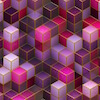### Bundles of Cubes

##### Age 7 to 11 Challenge Level:

Watch this animation. What do you notice? What happens when you try more or fewer cubes in a bundle?### Swimming Pool Tiles

##### Age 7 to 11 Challenge Level:

This activity creates an opportunity to explore all kinds of number-related patterns.### Diagonal in a Spiral

##### Age 7 to 11 Challenge Level:

Investigate the totals you get when adding numbers on the diagonal of this pattern in threes.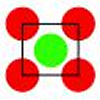### Centred Squares

##### Age 7 to 11 Challenge Level:

This challenge, written for the Young Mathematicians' Award, invites you to explore 'centred squares'.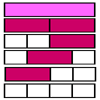### Rods and Rods

##### Age 7 to 11 Challenge Level:

Using only the red and white rods, how many different ways are there to make up the other colours of rod?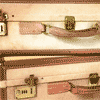### Unlocking the Case

##### Age 7 to 11 Challenge Level:

A case is found with a combination lock. There is one clue about the number needed to open the case. Can you find the number and open the case?### Four Coloured Lights

##### Age 11 to 14 Challenge Level:

Imagine a machine with four coloured lights which respond to different rules. Can you find the smallest possible number which will make all four colours light up?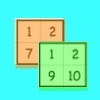### Sorting the Numbers

##### Age 5 to 11 Challenge Level:

Complete these two jigsaws then put one on top of the other. What happens when you add the 'touching' numbers? What happens when you change the position of the jigsaws?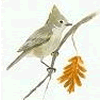### Birds in the Garden

##### Age 5 to 11 Challenge Level:

This activity asks you to collect information about the birds you see in the garden. Are there patterns in the data or do the birds seem to visit randomly?### Sorting Numbers

##### Age 5 to 7 Challenge Level:

Use the interactivity to sort these numbers into sets. Can you give each set a name?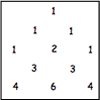### Investigating Pascal's Triangle

##### Age 7 to 14 Challenge Level:

In this investigation, we look at Pascal's Triangle in a slightly different way - rotated and with the top line of ones taken off.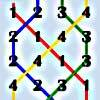### Oranges and Lemons, Say the Bells of St Clement's

##### Age 11 to 14 Challenge Level:

Bellringers have a special way to write down the patterns they ring. Learn about these patterns and draw some of your own.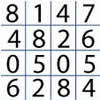### Tables Without Tens

##### Age 7 to 11 Challenge Level:

Investigate and explain the patterns that you see from recording just the units digits of numbers in the times tables.### Colour Building

##### Age 11 to 14 Challenge Level:

Using only the red and white rods, how many different ways are there to make up the other colours of rod?### Triangles Within Pentagons

##### Age 14 to 16 Challenge Level:

Show that all pentagonal numbers are one third of a triangular number.### Can You Find a Perfect Number?

##### Age 7 to 14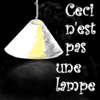### Shedding Some Light

##### Age 7 to 11 Challenge Level:

Make an estimate of how many light fittings you can see. Was your estimate a good one? How can you decide?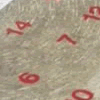### All Seated

##### Age 7 to 11 Challenge Level:

Look carefully at the numbers. What do you notice? Can you make another square using the numbers 1 to 16, that displays the same properties?### Pattern Power

##### Age 5 to 14

Mathematics is the study of patterns. Studying pattern is an opportunity to observe, hypothesise, experiment, discover and create.### Squares, Squares and More Squares

##### Age 11 to 14 Challenge Level:

Can you dissect a square into: 4, 7, 10, 13... other squares? 6, 9, 12, 15... other squares? 8, 11, 14... other squares?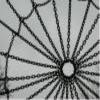### Taking Steps

##### Age 7 to 11 Challenge Level:

In each of the pictures the invitation is for you to: Count what you see. Identify how you think the pattern would continue.### Lastly - Well

##### Age 11 to 14 Challenge Level:

What are the last two digits of 2^(2^2003)?### A One in Seven Chance

##### Age 11 to 14 Challenge Level:

What is the remainder when 2^{164}is divided by 7?### Rolling Coins

##### Age 14 to 16 Challenge Level:

A blue coin rolls round two yellow coins which touch. The coins are the same size. How many revolutions does the blue coin make when it rolls all the way round the yellow coins? Investigate for a. . . .### Top-heavy Pyramids

##### Age 11 to 14 Challenge Level:

Use the numbers in the box below to make the base of a top-heavy pyramid whose top number is 200.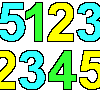### Sept03 Sept03 Sept03

##### Age 7 to 11 Challenge Level:

This number has 903 digits. What is the sum of all 903 digits?### Hidden Rectangles

##### Age 11 to 14 Challenge Level:

Rectangles are considered different if they vary in size or have different locations. How many different rectangles can be drawn on a chessboard?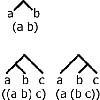### Counting Binary Ops

##### Age 14 to 16 Challenge Level:

How many ways can the terms in an ordered list be combined by repeating a single binary operation. Show that for 4 terms there are 5 cases and find the number of cases for 5 terms and 6 terms.### Magic Squares II

##### Age 14 to 18

An article which gives an account of some properties of magic squares.### Magic Squares

##### Age 14 to 18

An account of some magic squares and their properties and and how to construct them for yourself.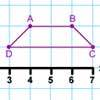### Transformation Tease

##### Age 7 to 11 Challenge Level:

What are the coordinates of this shape after it has been transformed in the ways described? Compare these with the original coordinates. What do you notice about the numbers?### Back to Basics

##### Age 14 to 16 Challenge Level:

Find b where 3723(base 10) = 123(base b).### Power Crazy

##### Age 11 to 14 Challenge Level:

What can you say about the values of n that make $7^n + 3^n$ a multiple of 10? Are there other pairs of integers between 1 and 10 which have similar properties?### Small Change

##### Age 11 to 14 Challenge Level:

In how many ways can a pound (value 100 pence) be changed into some combination of 1, 2, 5, 10, 20 and 50 pence coins?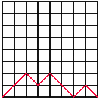##### Age 14 to 16 Challenge Level:

A walk is made up of diagonal steps from left to right, starting at the origin and ending on the x-axis. How many paths are there for 4 steps, for 6 steps, for 8 steps?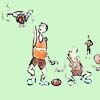### One Basket or Group Photo

##### Age 7 to 18 Challenge Level:

Libby Jared helped to set up NRICH and this is one of her favourite problems. It's a problem suitable for a wide age range and best tackled practically.### Sum Equals Product

##### Age 11 to 14 Challenge Level:

The sum of the numbers 4 and 1 [1/3] is the same as the product of 4 and 1 [1/3]; that is to say 4 + 1 [1/3] = 4 � 1 [1/3]. What other numbers have the sum equal to the product and can this be so for. . . .### Like Powers

##### Age 11 to 14 Challenge Level:

Investigate $1^n + 19^n + 20^n + 51^n + 57^n + 80^n + 82^n$ and $2^n + 12^n + 31^n + 40^n + 69^n + 71^n + 85^n$ for different values of n.### Pinned Squares

##### Age 14 to 16 Challenge Level:

What is the total number of squares that can be made on a 5 by 5 geoboard?